PHP弱类型

参考：

PHP弱类型安全问题总结 | 乘物游心

PHP弱类型简介

• 在PHP中，可以进行以下的操作。
$param = 1;$param = array();
$param = "stringg"; • 弱类型的语言对变量的数据类型没有限制，你可以在任何地时候将变量赋值给任意的其他类型的变量，同时变量也可以转换成任意地其他类型的数据。 类型转换问题 • 类型转换是无法避免的问题。例如需要将GET或者是POST的参数转换为int类型，或者是两个变量不匹配的时候，PHP会自动地进行变量转换。但是PHP是一个弱类型的语言，导致在进行类型转换的时候会存在很多意想不到的问题。 比较操作符 类型转换 •$a==$b的比较中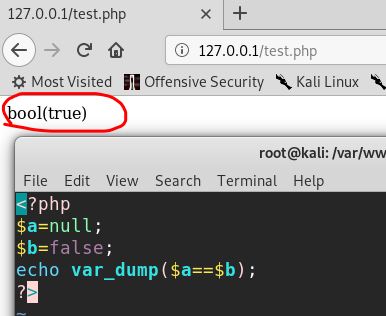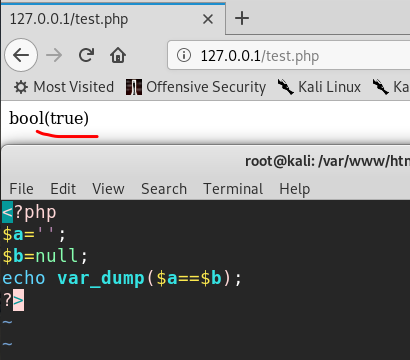• 这样的例子还有很多，这种比较都是相等。PHP会把类数值(如含有数字的字符串等)的字符串转换为数值进行比较，如果参数是字符串，则返回字符串中第一个不是数字的字符之前的数字串所代表的整数值 • 使用比较操作符的时候也存在类型转换的问题，如下：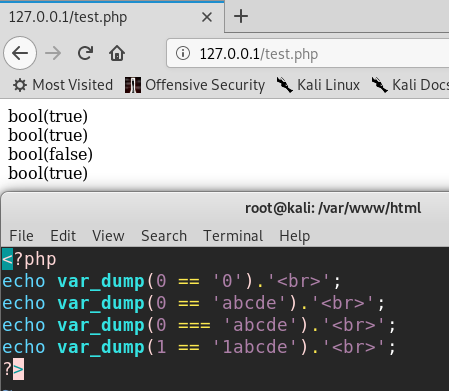• 不同类型的变量进行比较的时候就会存在变量转换的问题，在转换之后就有可能会存在问题。 • 当一个字符串当作一个数值来取值，其结果和类型如下: • 如果该字符串没有包含.eE并且其数值值在整型的范围之内，该字符串被当作int来取值，其他所有情况下都被作为float来取值，该字符串的开始部分决定了它的值 • 如果该字符串以合法的数值开始，则使用该数值，否则其值为0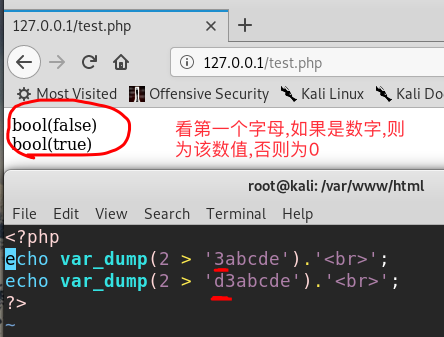• 这里需要注意一下，第二个条件是===，和==是有点区别的： • ===是恒等计算符 同时检查表达式的值与类型,可以理解为先检查类型再检查值 • ==是比较运算符号 不会检查条件式的表达式的类型,如果类型不同的进行比较，其会将类型转换成相同的再进行比较 Hash比较 kali中输入如下代码验证：php -r 'var_dump("00e0345" == "0");'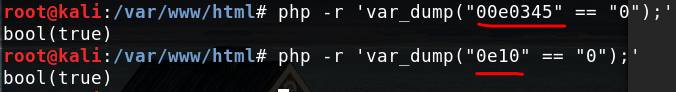• 除了以上的这种方式之外在进行hash比较的时候也会存在问题。php在处理hash字符串的时候会用到!=,==来进行hash比较，如果hash值以0e开头，后边都是数字，再与数字比较，就会被解释成 科学记数法:0*10^n还是为0，就会被判断相等,如下： "0e132456789"=="0e7124511451155" // true "0e123456abc"=="0e1dddada" // false "0e1234abc"=="0" // false • 当全是数字的时候，宽松的比较会执行尽力模式，如0e12345678会被解释成0*10^12345678, 除了e外不全是数字的时候就不会相等 例题 <?php error_reporting(0);$flag = 'flag{test}';
if  ("POST" == $_SERVER['REQUEST_METHOD']) {$password = $_POST['password']; if (0 >= preg_match('/^[[:graph:]]{12,}$/', $password)) { echo 'Wrong Format'; exit; } while (TRUE) {$reg = '/([[:punct:]]+|[[:digit:]]+|[[:upper:]]+|[[:lower:]]+)/';
if (6 > preg_match_all($reg,$password, $arr)) break;$c = 0;
$ps = array('punct', 'digit', 'upper', 'lower'); foreach ($ps as $pt) { if (preg_match("/[[:$pt:]]+/", $password))$c += 1;
}

string转int：intval()函数。
• 对于这个函数，可以先看2个例子。
var_dump(intval('2'))    //2
var_dump(intval('3abcd'))    //3
var_dump(intval('abcd'))    //0
• 说明intval()转换的时候，会将从字符串的开始进行转换直到遇到一个非数字的字符。即使出现无法转换的字符串，intval()不会报错而是返回0
• 同时，程序员在编程的时候也不应该使用如下的这段代码：
if(intval($a)>1000) { mysql_query("select * from news where id=".$a)
}

$temp = "1337a"; is_numeric($temp)?die("nope"):NULL;
if($temp>1336){ echo$flag;
}
?>
• 代码中先将变量放到is_numeric函数中判断，如果数字或数字字符串则返回true，否咋返回false。然后一个判断，如果temp大于1336则显示flag。这里用到了PHP弱类型的一个特性，当一个整型和一个其他类型行比较的时候，会先把其他类型intval再比。如果输入一个1337a这样的字符串，在is_numeric中返回true，然后在比较时被转换成数字1337，这样就绕过判断输出flag。

bool欺骗

$json_str = '{"user":true,"pass":true}';$data = json_decode($json_str,true); if ($data['user'] == 'admin' && $data['pass']=='secirity') { print_r('logined in as bool'."\n"); } 运行结果： root@kali:/var/www# php /root/php/hash.php logined in as bool unserialize$unserialize_str = 'a:2:{s:4:"user";b:1;s:4:"pass";b:1;}';
$data_unserialize = unserialize($unserialize_str);
if ($data_unserialize['user'] == 'admin' &&$data_unserialize['pass']=='secirity')
{
}

root@kali:/var/www# php /root/php/hash.php

bool类型的true跟任意字符串可以弱类型相等。因此我们可以构造bool类型的序列化数据 ，无论比较的值是什么，结果都为true。（a代表arrays代表stringb代表bool，而数字代表个数/长度）

数字转换欺骗

$user_id = ($_POST['user_id']);
if ($user_id == "1") {$user_id = (int)($user_id); #$user_id = intval($user_id);$qry = "SELECT * FROM users WHERE user_id='$user_id';"; }$result = mysql_query($qry) or die('<pre>' . mysql_error() . '</pre>' ); print_r(mysql_fetch_row($result));

user_id=0.999999999999999999999发送出去得到结果如下：

Array
(
 => 0
 => lxx'
 =>
 =>
 =>
 =>
)

if ($_POST['uid'] != 1) {$res = $db->query("SELECT * FROM user WHERE uid=%d", (int)$_POST['uid']);
mail(...);
} else {
}

{
$db->query("select * from user where qq =$qq")
}

内置函数的参数的松散性

md5()

$array1[] = array( "foo" => "bar", "bar" => "foo", );$array2 = array("foo", "bar", "hello", "world");
var_dump(md5($array1)==var_dump($array2));    //true

PHP手册中的md5()函数的描述是string md5 ( string $str [, bool$raw_output = false ] )md5()中的需要是一个string类型的参数。但是当你传递一个array时，md5()不会报错，只是会无法正确地求出array的md5值，md5的结果都是null这样就会导致任意2个array的md5值都会相等

例题

<?php
error_reporting(0);
$flag = 'flag{test}'; if (isset($_GET['username']) and isset($_GET['password'])) { if ($_GET['username'] == $_GET['password']) print 'Your password can not be your username.'; else if (md5($_GET['username']) === md5($_GET['password'])) die('Flag: '.$flag);
else
}
?>

strcmp()

strcmp()函数在PHP官方手册中的描述是int strcmp ( string $str1 , string$str2 ),需要给strcmp()传递2个string类型的参数。如果str1小于str2,返回-1，相等返回0，否则返回1。strcmp函数比较字符串的本质将两个变量转换为ascii，然后进行减法运算，然后根据运算结果来决定返回值。 如果传入给出strcmp()的参数是数组呢？

$array=[1,2,3]; var_dump(strcmp($array,'123')); //结果是null

例题
<?php
if (isset($_GET['password'])) { if (strcmp($_GET['password'], $flag) == 0) die('Flag: '.$flag);
else
}
?>

switch()

• 如果switch是数字类型的case的判断时，switch会将其中的参数转换为int类型。如下：
$i ="2abc"; switch ($i) {
case 0:
case 1:
case 2:
echo "i is less than 3 but not negative";
break;
case 3:
echo "i is 3";
}

in_array — 检查数组中是否存在某个值 参数
needle 待搜索的值。

haystack 这个数组。
strict 如果第三个参数 strict 的值为 TRUE 则 in_array() 函数还会检查 needle 的类型是否和 haystack 中的相同。

$array=[0,1,2,'3']; var_dump(in_array('abc',$array));  //true
var_dump(in_array('1bc', \$array));    //true
// 它遍历了array的每个值，并且作"=="比较（“当设置了strict 用===”）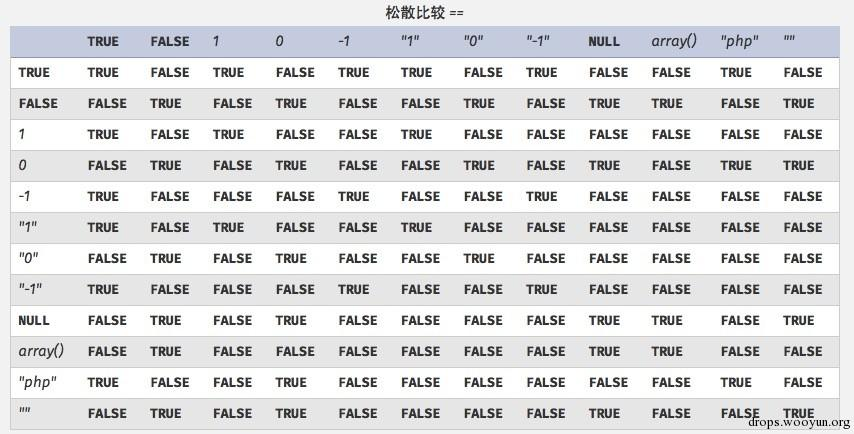上一篇Web Server解析漏洞
1. Apache解析漏洞1.1. 未知后缀解析漏洞1.1.1. 影响版本 Apache 1.x Apache 2.x 1.1.2. 原理 Apache对于⽂件名的解析是从后往前解析的, 直到遇⻅⼀个Apache认识的⽂件类型为⽌. 比如
2019-04-27web源码泄露总结

2019-03-10
目录